# Go Math Grade 3 Answer Key Chapter 8 Understand Fractions Assessment Test

Go Math Grade 3 Answer Key Chapter 8 contains all the topics of chapter 8 which helps to test the student’s knowledge. By this assessment test students can check their knowledge. This assessment test are also helpful for the teachers to know how much a student understood the topics.

### Go Math Grade 3 Answer Key Chapter 8 Understand Fractions Assessment Test

With this chapter, students can improve their math skills by referring to the Go Math Grade 3 Answer Key Chapter 8 Understand Fractions Assessment Test. With the help of this Go Math Grade 3 Assessment Test Answer Key, you can score good marks in the examination.

Chapter 8: Understand Fractions Assessment Test

### Test – Page 1 – Page No. 81

Question 1.
Each shape is divided into equal parts. Select the shapes that show fourths. Mark all that apply.
Options:
a.b.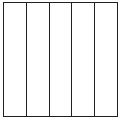c.d.Explanation: a,c,d shapes that show fourths. As a,c,d shapes are divided into four parts.

Question 2.
What fraction names the shaded part of the shape?Options:
a. 3 eighths
b. 5 eighths
c. 6 eighths
d. 8 eighths

Explanation: The given shape is divided into 8 equal parts in which 5 parts are shaded, so fraction name of the shaded part is 5 eights. i.e. 5/8.

Question 3.
The shaded part of the model shows what part of a garden is planted with peas. What fraction names the shaded part? Explain how you know how to write the fraction.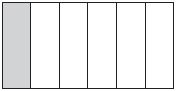$$\frac{□}{□}$$

Answer: $$\frac{1}{6}$$.

Explanation: Given a model of the garden shows 6 equal parts in it. Out of which, 1 part is where peas are planted is shaded. Therefore, fraction names of the shaded part is $$\frac{1}{6}$$.

### Test – Page 2 – Page No. 82

Question 4.
What fraction names point A on the number line?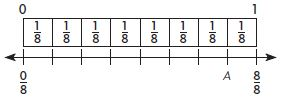$$\frac{□}{□}$$

Answer: $$\frac{7}{8}$$

Explanation: Given the number line is divided into 8 parts, A is at 7th position. Therefore, the fraction name of point A on the number line is $$\frac{7}{8}$$.

Question 5.
Kwan divided this circle into equal parts. Circle the word that makes the sentence true.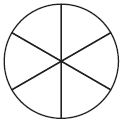The circle is divided into_________

Explanation: Kwan divided the circle into six parts. Therefore the answer is Sixths.

Question 6.
Sophie uses 16 beads to make a necklace. One fourth of the beads are purple. How many of the beads are purple?

Explanation:
Total no of beads used by Sophie is = 16
a portion of purple beads used = ¼
Therefore total no of purple beads = 16× ¼ = 4 beads.

Question 7.
Four brothers work together to mow 3 equal-sized fields. Each brother mows the same amount.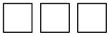How much does each brother mow? Mark all that apply.
Options:
a. 4 thirds of a field
b. 1 whole and 1 third of a field
c. 3 fourths of a field
d. 2 thirds of a field
e. 1 fourth of a field

Explanation:
No of persons to work = 4
total no of fields = 3
So dividing 3 equal-sized fields 4 equal parts = ¾
Therefore each person gets ¾ of the field as their share to mow.

### Test – Page 3 – Page No. 83

Question 8.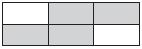Select one number from each column to show the part of the model Bailey shaded.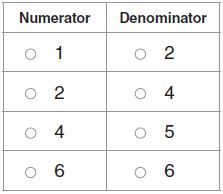Answer: Numerator – 4, Denominator – 6.

Explanation: Given the model shows 6 equal parts in which Bailey shaded 4 parts. Therefore, the fractional part Bailey shaded is 4/6. From the fraction Numerator is 4, Denominator is 6.

Question 9.
Jayson baked a pan of cornbread for a family dinner. He cut the cornbread into equal size pieces. At the end of the dinner, there were 2 pieces left. Explain how you find the number of pieces in the whole pan of cornbread if Jayson told you that 16 of the pan was left. Use a drawing to show your work.
_____ pieces

Explanation:No of bread pieces left after dinner = 2
Considering 1/6 of the pan was left, we draw 6 ovals since the denominator is 6. Since 2 pieces are 1/6 of the pan, we represent them two circles in each oval. Therefore total no of pieces in the whole pan of cornbread are = 6 × 2 = 12.

### Test – Page 4 – Page No. 84

Question 10.
The model shows one whole. What fraction of the model is NOT shaded?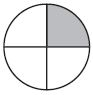$$\frac{□}{□}$$

Answer: $$\frac{3}{4}$$ of the model is not shaded.

Explanation: Given model is a circle that is divided into 4 parts. Out of which 1 part is shaded. Thus $$\frac{3}{4}$$ of the model is not shaded.

Question 11.
Michael replaced $$\frac{1}{3}$$ of the buttons on his coat. If he replaced a total of 3 buttons, how many buttons are on his coat? Show your work.
______ buttons

Explanation:
Let’s consider the total no of buttons be X
Given that Michael replaced 1/3 of his coat buttons which are 3 in number
which means 1/3 × X = 3
therefore X = 3×3 = 9
So the total no of buttons on his coat are 9.

Question 12.
Four teachers share 3 gallons of paint equally. How much paint does each teacher get?_____ fourths

Explanation:
No of teachers to share paint = 4
the total amount of paint = 3 gallons
So dividing 3 gallons of paint into 4 equal parts = ¾
Therefore each teacher gets ¾ of paint as their share.

Question 13.
Each shape is 1 whole.For numbers 13a–13e, choose Yes or No to show whether the number names the parts that are shaded.
a. 3
i. yes
ii. no

Explanation: The total 4 models are shaded.

Question 13.
b. 4
i. yes
ii. no

Explanation: The 4 models are shaded.

Question 13.
c. $$\frac{12}{3}$$
i. yes
ii. no

Explanation: $$\frac{12}{3}$$ means 4 parts, which represents 4 shaded parts/model.

Question 13.
d. $$\frac{12}{4}$$
i. yes
ii. no

Explanation: $$\frac{12}{4}$$ means 3 parts, which represents 3 shaded parts/model. So answer is no.

Question 13.
e. $$\frac{3}{12}$$
i. yes
ii. no

Explanation: $$\frac{3}{12}$$ means $$\frac{1}{4}$$ part is shaded. So answer is no.

### Test – Page 5 – Page No. 85

Question 14.
A store sold 6 fruit trees. Five of the trees were apple trees. What fraction of the trees were apple trees?
$$\frac{□}{□}$$

Answer: $$\frac{5}{6}$$

Explanation:
Given that total of 6 trees were sold
no of apple trees are 5
Therefore fraction of apple trees out of total sold trees are = No of apple trees / Total no of trees
= $$\frac{5}{6}$$.

Question 15.
Christina and Erin made vegetable trays for a neighborhood picnic.
Part A
Christina put 15 vegetables on her tray. If $$\frac{1}{5}$$ of the vegetables were carrots, how many carrots were on the tray? Make a drawing to show your work.
_____ carrots

Explanation:Total vegetables on Christina tray = 15
part of carrots on the tray  = 1/5
Total of carrots = (Total no of vegetables × part of the carrots)
= 15 × 1/5 = 3 carrots.

Question 15.
Part B
Erin put 20 vegetables on her tray. If $$\frac{1}{4}$$ of them were carrots, how many carrots were on Christina’s and Erin’s trays? Explain how you found your answer.
_____ carrots

Explanation:
No of carrots in Christina’s tray = 3
Total vegetables on Erin’s tray = 20
part of carrots on the tray  = 1/4
Total of carrots = (Total no of vegetables × part of the carrots)
= 20 × 1/4
= 5 carrots.
Therefore total no of carrots on both of their trays = 5 + 3 = 8 carrots

Question 16.
Maria has 8 tulip bulbs. She gives 5 of the tulip bulbs to her neighbor. What fraction of her tulip bulbs does she give to her neighbor?
$$\frac{□}{□}$$ tulip bulbs

Answer: $$\frac{5}{8}$$

Explanation:
Total no of tulip bulbs Maria has = 8
No of tuple bulbs she gave to neighbor = 5
Therefore fraction of tuple bulbs she gave =$$\frac{5}{8}$$.

### Test – Page 6 – Page No. 86

Question 17.
Lucy rode her bike around the block 4 times for a total of 1 mile yesterday. Today she wants to ride her bike $$\frac{3}{4}$$ of a mile. How many times will she need to ride her bike around the block?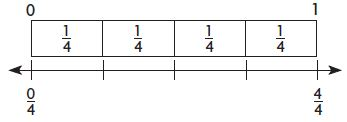______ times

Explanation:
No of times Lucy rode to complete 1 mile = 4
So in one time, she completes ¼ mile
Therefore to complete ¾ mile, she has to complete = ¼ + ¼ + ¼, which is 3 times

Question 18.
Jackson colored some shapes.Select one number from each column to show a fraction greater than 1 that names the parts Jackson colored.Answer: Numerator – 6, Denominator – 2

Explanation: As there are 6 halves that are shaded and the fraction greater than 1 is $$\frac{6}{2}$$.

Question 19.
Samira ran around a park loop that was $$\frac{1}{3}$$ mile long. She ran around the loop 9 times. Samira says she ran $$\frac{9}{3}$$ miles. Her brother Amal says she ran 3 miles. Who is correct? Use words and drawings to explain how you know.
_________

Explanation:
The distance of the loop is = $$\frac{1}{3}$$ mile
Samira ran around nine times = 9 × $$\frac{1}{3}$$
=$$\frac{9}{3}$$
= 3miles

So both are correct.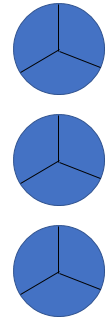Each circle represents a park where each shaded part we considered as 1/3 mile. Each circle can be completed in 3 rounds, therefore running nine times covers all the three circles = 9 ×$$\frac{1}{3}$$
= $$\frac{9}{3}$$.
= 3miles.

Conclusion:

In this Grade 3 Chapter 8 assessment test students can test their knowledge and can know their performing skills. Every question was explained in a brief way that students can catch the logic easily.

Scroll to Top